Editing [River Survey Data]¶

[River Survey Data] is a type of Geographic Data] that is based on actual cross-sectional survey data of a river. Generally, [River Survey Data] is used as the elevation information for a river and its environs.

Figure 134 shows an example of [River Survey Data].

Selecting the river transverse line¶

The river transverse lines need to be selected prior to any operations explained in the following sections, except for displaying elevation colormap. In this section, the operation to select the river transverse line is explained.

To select river transverse lines, left drag on the canvas to make a rectangle (black line) (Figure 135). When you releasing the mouse left button, the river transverse lines whose river center point (blue dot) is in the rectangle (Figure 136) are selected. The selected river transverse lines are drawn with thicker line.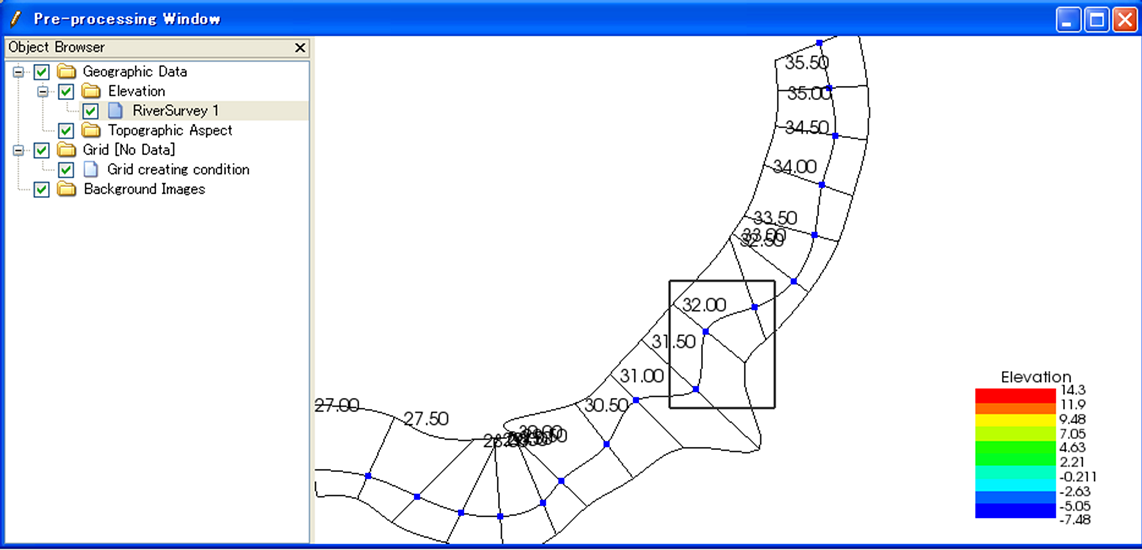Figure 135 The [Pre-processing Window] when a user is left dragging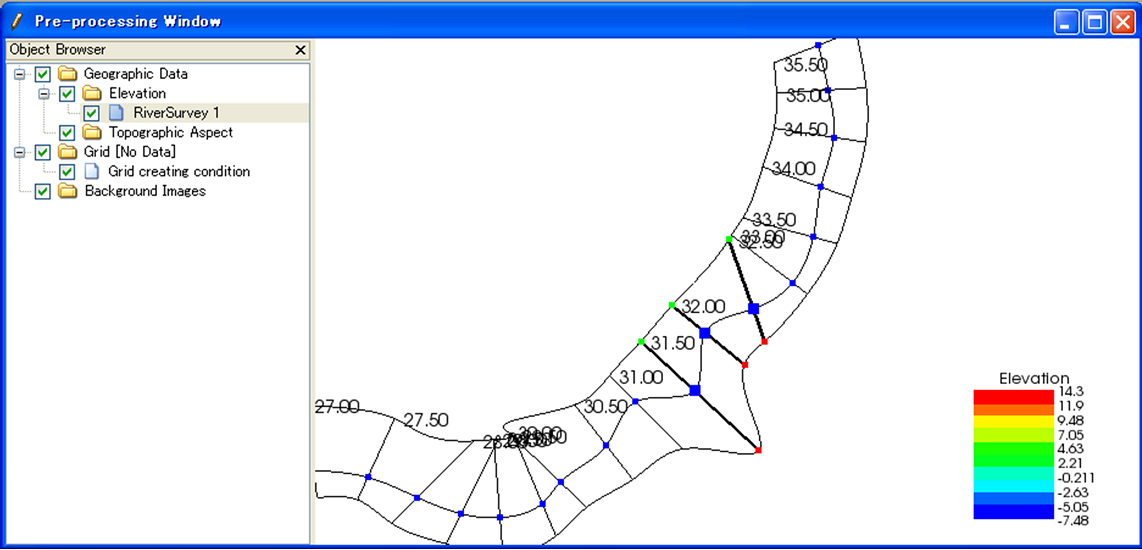Figure 136 The [Pre-processing Window] after selecting river transverse lines

[Display Cross-section] (C)¶

Description: Displays the cross-section window.

Prior to this operation, you need to select the river transverse line for which you want to open cross-section window. Figure 137 shows an example of the cross-section window.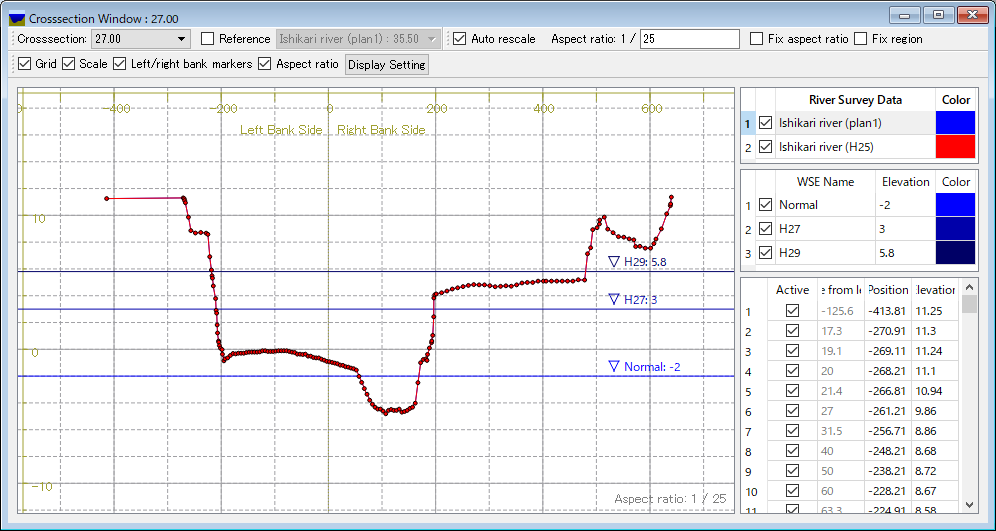Figure 137 The river cross-section window

For operations in the cross-section window, refer to Operation in the River Cross-section Window.

[Insert Upstream Side] (B) / [Insert Downstream Side] (A)¶

Description: Inserts a new river transverse line upstream (or downstream) from the selected river transverse line.

Prior to this operation, you need to select a river transverse line.

When you select one of these, the [Insert Transverse Line] dialog (Figure 138) will open. Set [River Center Point Coordinates] and [Cross-section Information] and click on [OK].

• [Center Point Coordinates]:
• If you have chosen [Mouse Click], click on the canvas to set the coordinates.
• If you have chosen [Coordinate Values], input the coordinate values in the boxes.
• If you have chosen [Ratio between Back and (0-1)], set the ratio between 0 and 1 to locate the center point on the spline curve. The curve is made by smoothly linking the points before and after the added point.
• [Cross-section Information]:
• If you have chosen [Insert Three Points of Elevation 0], then input the cross-section data of the center, left bank and right bank while securing sufficient distance between each.
• If you have chosen [Copy Next River Transverse Line], copy the cross-section data of the river transverse line specified in the combo box.
• If you have chosen [Create Cross-section of Back and Forth], the cross-section data are made by interpolating the cross-section data of transverse lines immediately upstream/downstream from the transverse line that is to be inserted.

[Move] (M)¶

Description: Moves the selected river transverse lines. Figure 139 shows an example.

You can do this operation against multiple transverse lines at a time.Figure 139 Example of moving a river transverse line

When you select [Move], the [Move Transverse Line] dialog (Figure 140) will open. Select either [Center Point Coordinates] or [Transfer Quantity] and click on [OK].

You can also move the river transverse lines with mouse operation. When the mouse cursor is near the center point of the selected river transverse line, the cursor changes to that in Figure 141. Now you can move the selected river transverse lines by left dragging.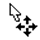Figure 141 Mouse cursor you can move river transverse lines

[Rotate] (R)¶

Description: Rotates the selected river transverse line. Figure 142 shows an example.

You can do this operation against only one transverse line at a time.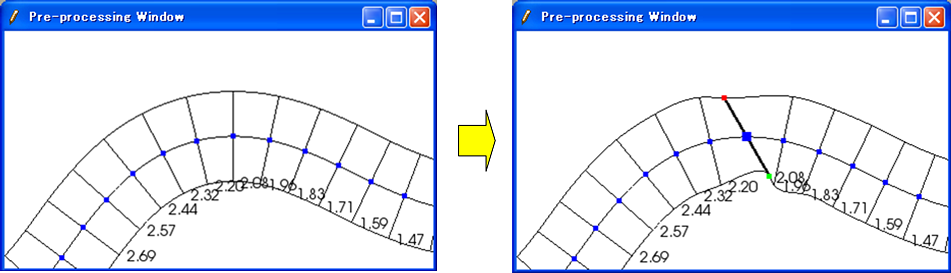Figure 142 Example of rotating a river transverse line

When you select [Rotate], the [Rotate Transverse Line] (Figure 143) dialog will open. Set either [Relative Angle] or [Increment Angle] and click on [OK]. (Positive direction: counterclockwise; unit: degree)

You can also rotate the river transverse lines with mouse operation. When the mouse cursor is near to the left (or right) bank of the selected river transverse line, the cursor changes to that in Figure 144. Now you can rotate the selected river transverse lines by left dragging.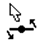Figure 144 Mouse cursor shape when you can rotate a river transverse line

[Shift Center] (H)¶

Description: Shifts the selected center point of the river transverse line to the left (or right) bank side. By this operation, the location of the center point shifts but the cross-section data do not change. Figure 145 shows an example.

You can do this operation against multiple transverse lines at a time.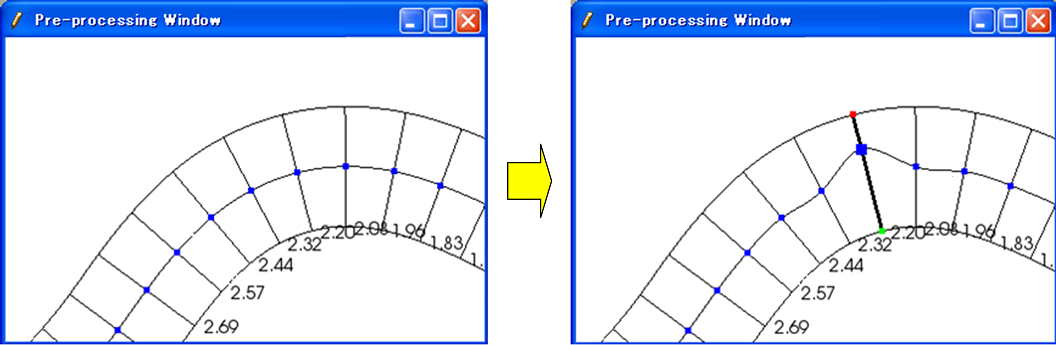Figure 145 Example of operation to shift the center point

When you select [Shift Center], the [Shift River Center] dialog (Figure 146) will open. Set [Transfer Quantity] and click on [OK].

You can also shift the center point of the river transverse line on canvas with mouse operation. When the mouse cursor is near the center point of the selected river transverse line while the Shift key is held down, the cursor changes to that in Figure 147. Now, you can shift the selected center point of the river transverse line with left dragging.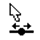Figure 147 Mouse cursor shape when you can shift the center point of the river transverse line

[Extend Horizontally] (X)¶

Description: Extends/Shortens the river transverse lines. Figure 148 shows an example.

You can do this operation against multiple transverse lines at a time.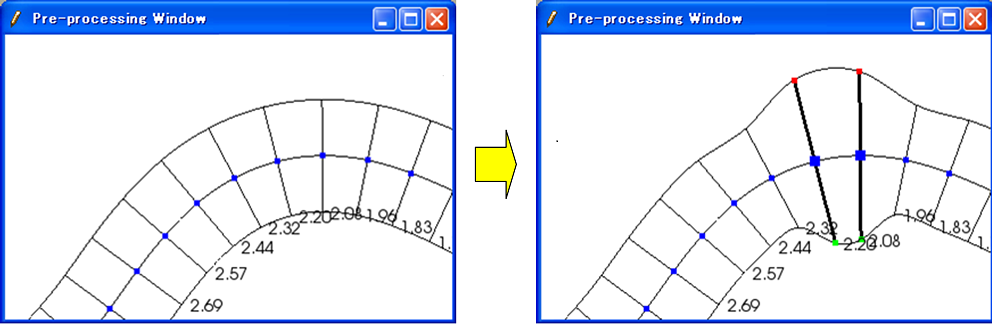Figure 148 Example of extending the river transverse lines

When you select [Extend Horizontally], the [Extend Transverse Line] dialog will open (Figure 149). Select from among [Set Distance between River Center and Left Bank], [Increment] and [Extension Ratio], set the value and click on [OK].

[Delete Cross Section] (T)¶

Description: Deletes the selected river transverse lines. Figure 150 shows an example.

You can do this operation against multiple transverse lines at a time.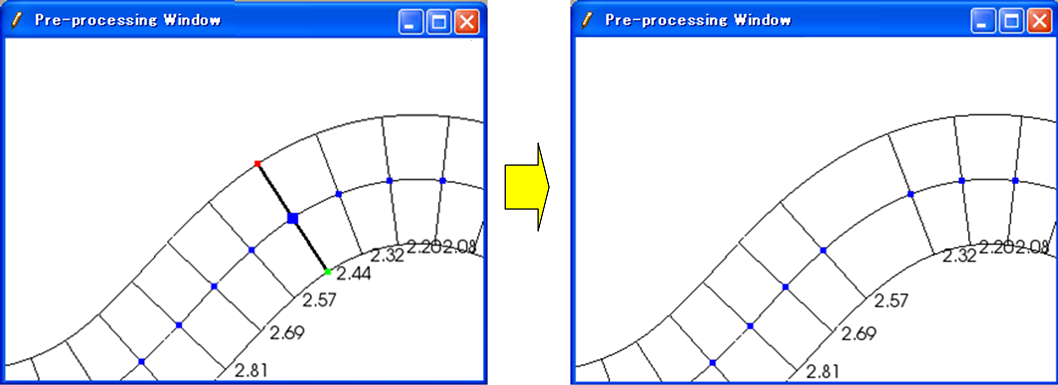Figure 150 Example of deleting a river transverse line

[Rename Cross Section] (E)¶

Description: Renames the selected river transverse line.

You can do this operation against only one transverse line at a time.

When you select this, the [Rename Transverse Line] dialog (Figure 151) will open. Set a new name and click on [OK].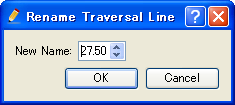Figure 151 Example of [Rename Transverse Line] dialog

[Add Extension Line] (L) / [Add Extension Line] (R)¶

Description: Adds a Left Bank Extension Line or Right Bank Extension Line to the selected river transverse line. Figure 152 shows an example.

You can do this operation against only one transverse line at a time.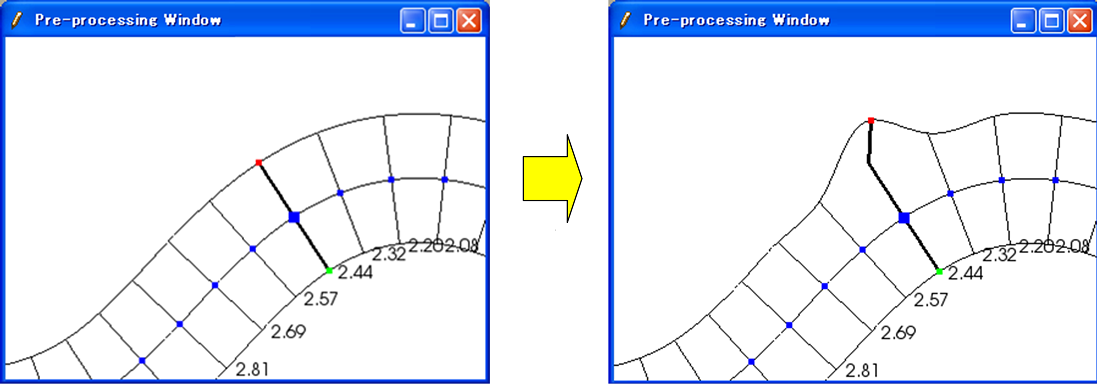Figure 152 Example of adding a Extension Line to the selected river transverse line

When you select [Add Left Bank Extension Line], the [Add Extension Line] dialog (Figure 153) will open. When you select [Mouse Click], click on the point to which you want to extend the river transverse line, or when you select [Coordinates], input the coordinates of the point to which you want to extend the river transverse line. Then click on [OK].

[Remove Left Bank Extension Line] (V) / [Remove Right Bank Extension Line] (O)¶

Description: Deletes a Left Bank Extension Line/Right Bank Extension Line from the selected river transverse line. Figure 154 shows an example.

You can do this operation against only one transverse line at a time. This operation is possible against a river transverse line which has been added the Left Bank Extension Line or Right Bank Extension Line.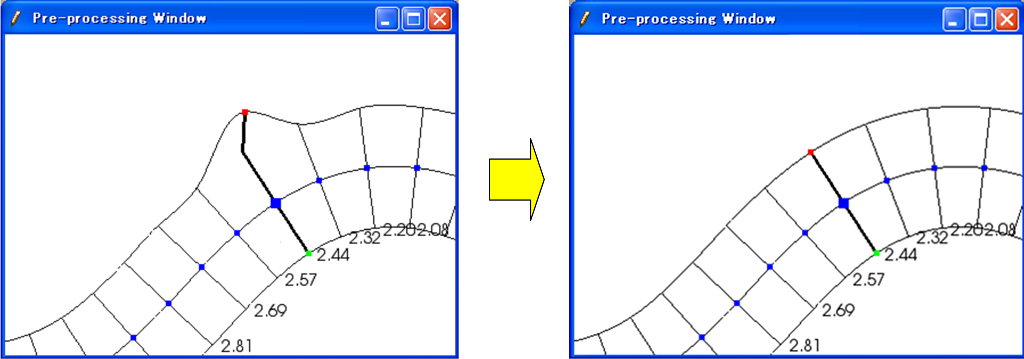Figure 154 Example of the operation for deleting a Extension Line

[Display Setting]¶

Description: Edits display setting of [River Survey Data]. Figure 155 shows an example.Figure 155 Example of changing [River Survey Data] display setting

When you select [Display Setting], the [Display Setting] dialog (Figure 156) will open. When [Visible] check box inside [Background Color] group box is checked, [River Survey Data] region background is painted. When [Visible] check box inside [Crosssection Lines] group box is checked, the cross-section lines are drawn just below the transverse line, with the Z-scale and color in that group box.

[Interpolation Mode]¶

Description: Switches the Interpolation Mode (“Spline” or “Linear Curve”).

Figure 157 shows an example of Spline Interpolation Mode. Figure 158 shows an example of Linear Curve Interpolation Mode.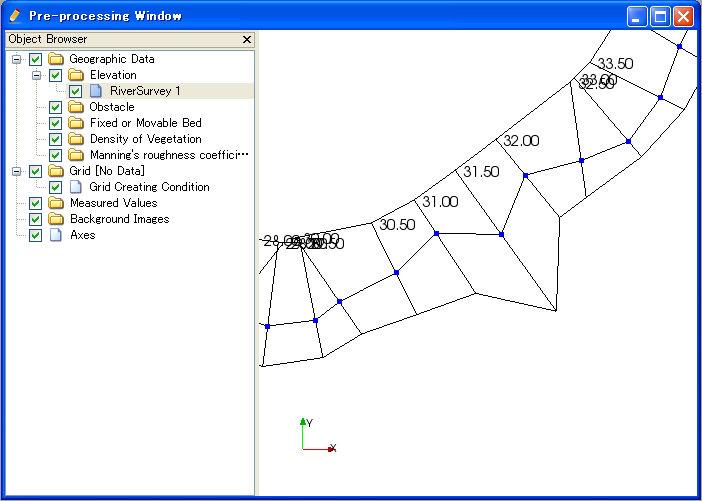Figure 158 Example of the [Interpolation Mode (Linear Curve)]

Operation in the River Cross-section Window¶

Description: Displays the cross-section of a river transverse line. The window is used to edit elevation information. Figure 159 shows an example of the River Cross-section Window.Figure 159 Example of the River Cross-section Window display

Table 11 shows the additional menu items for the River Cross-section Window. The additional menu items are shown between [Import] and [Simulation] when River Cross-section Window is active.

Table 11 The additional menu items for the River Cross-section Window
Elevation Point (A) [Activate] (A) Activates the selected elevation point.
[Inactivate] (I) Inactivates the selected elevation point.
[Inactivate using water elevation] Inactivate points that are outside of the positions where the elevation exceed water elevation for the first time.
[Edit cross section from the selected point] Edit cross section shape from the selected point.
[Move] (M) Moves the selected elevation point.
[Delete] (D) Deletes the selected elevation point.

[Activate] (A)¶

Description: Activates the selected elevation point. Figure 160 shows an example.

[Inactivate] (I)¶

Description: Inactivates the selected elevation point. Figure 161 shows an example.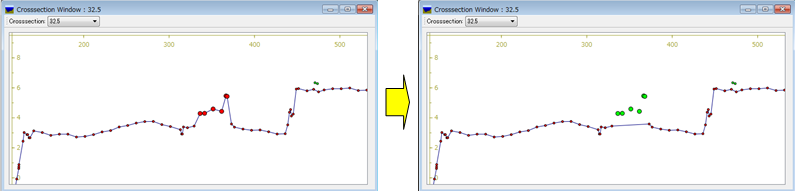Figure 161 Example of the inactivating an elevation point

[Inactivate using water elevation]¶

Description: Inactivate points that are outside of the positions where the elevation exceed water elevation for the first time.

When multiple water elevation data is loaded, a dialog to select the water elevation is shown.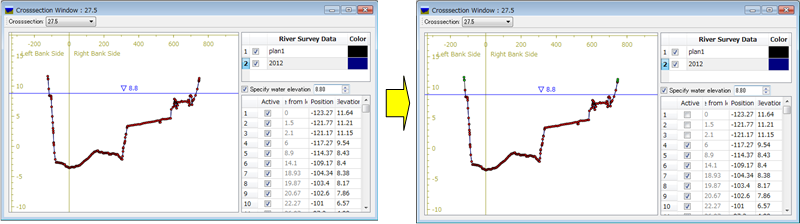Figure 162 Example of the operation for inactivate using water elevation

[Edit cross section from the selected point]¶

Edit the cross section shape from the selected point.

When you want to use this function, please select only one point on the cross section first.

After activating this function, when you move the mouse cursor, The line to preview the geometry shape after editing will be shown like in Figure 163. While editing, the horizontal and vertical length of the line, and the gradient will be shown for the line that is going to be created.

You can define the end point of the line by moving the mouse cursor, and execute the editing operation by left clicking.

You can finish editing operation by double clicking, or pressing Enter key.

The gradient values used in this function is selected from the values defined on [River Survey Data] tab.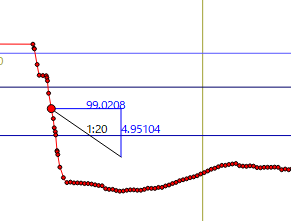Figure 163 Example of cross section editing operation by mouse

When you right click on the canvas while activating this function, [Edit from Dialog] menu will be shown. When you select this menu, the dialog in Figure 164 will be shown.

On this dialog, you can edit the cross section shape by inputting the distance and gradient etc.

By pressing [Apply] button, you can preview the cross section shape after editing.

By pressing [Edit Next] button, you can execute the editing operation, select the new point added by the operation, and continue editing. This function is useful when you want to edit the cross section shape continuously.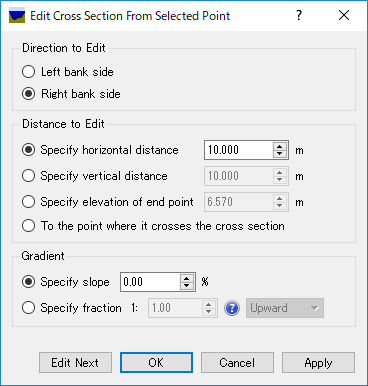Figure 164 Example of [Edit Cross Section From Selected Point] dialog

Using this function, you can easily create cross section shapes that is composed of line. Figure 165 shows an example.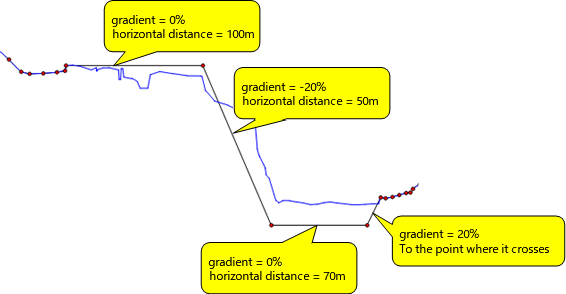Figure 165 Example of editing operation of cross section

[Move] (M)¶

Description: Moves the selected elevation point. Figure 166 shows an example.

When you select [Move], the [Move Elevation Point] dialog (Figure 167) will open. Set horizontal/vertical offset and click on [OK].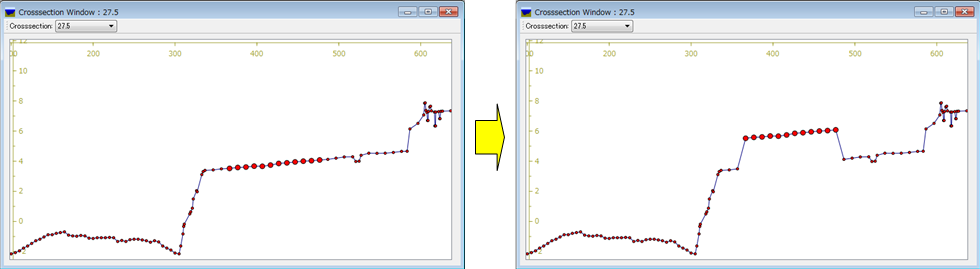Figure 166 Example of the operation for moving an elevation point

You can also move the elevation point on the canvas with a mouse operation. When the mouse cursor is near the selected elevation point, the cursor changes to an “open hand” cursor. Now you can move the selected elevation point by left dragging.

[Delete] (D)¶

Description: Deletes the selected elevation point. Figure 168 shows an example.